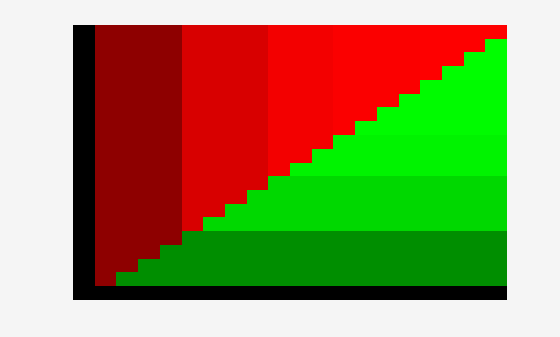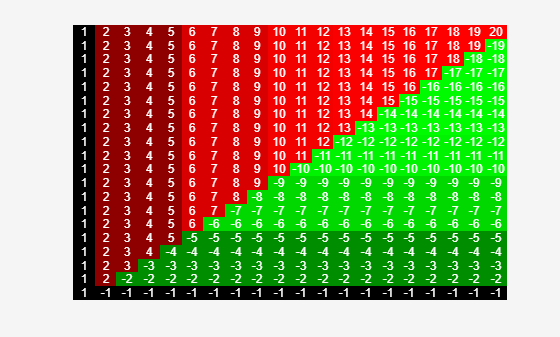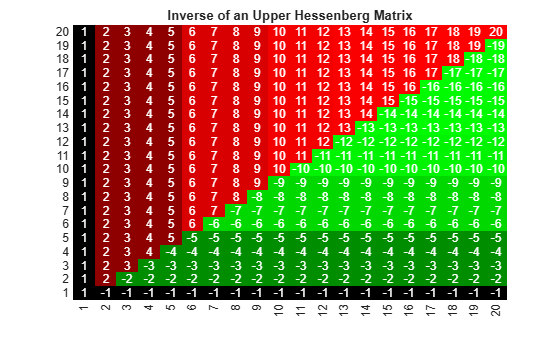# plot

Render heatmap or clustergram

## Syntax

``plot(hm_cg_object)``
``plot(hm_cg_object,hFig)``
``hAxes = plot(___)``

## Description

example

````plot(hm_cg_object)` renders a heatmap or clustergram of `hm_cg_object`. ```

example

````plot(hm_cg_object,hFig)` displays a heatmap or clustergram in a MATLAB® figure specified by the figure handle `hFig`.```
``` `hAxes = plot(___)` returns the handle to the axes of the heatmap or clustergram figure using any of the input argument combinations from the previous syntaxes. ```

## Examples

collapse all

Create a matrix of data.

`data = gallery('invhess',20);`

Display a 2-D color heatmap of the data.

`hmo = HeatMap(data);`
``` Standardize: '[column | row | {none}]' Symmetric: '[true | false].' DisplayRange: 'Scalar.' Colormap: [] ImputeFun: 'string -or- function handle -or- cell array' ColumnLabels: 'Cell array of strings, or an empty cell array' RowLabels: 'Cell array of strings, or an empty cell array' ColumnLabelsRotate: [] RowLabelsRotate: [] Annotate: '[on | {off}]' AnnotPrecision: [] AnnotColor: [] ColumnLabelsColor: 'A structure array.' RowLabelsColor: 'A structure array.' LabelsWithMarkers: '[true | false].' ColumnLabelsLocation: '[ top | {bottom} ]' RowLabelsLocation: '[ {left} | right ]' ```Display the data values in the heatmap.

```hmo.Annotate = true; view(hmo)```Use the `plot` function to display the heatmap in another figure specified by the figure handle `fH`.

```fH = figure; hA = plot(hmo,fH);```Use the returned axes handle `hA` to specify the axes properties.

```hA.Title.String = 'Inverse of an Upper Hessenberg Matrix'; hA.XTickLabelMode = 'auto'; hA.YTickLabelMode = 'auto';```## Input Arguments

collapse all

Heatmap or clustergram object, specified as a `HeatMap` object or `clustergram` object.

Handle to a figure to display the heatmap or clustergram, specified as a figure handle.

## Output Arguments

collapse all

Axes of the heatmap or clustergram figure, returned as an `axes` object.